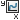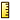Results PropertyManager (Motion Analysis only)

You can calculate and plot Motion Analysis results such as forces and displacements in the Results PropertyManager.

To open the Results PropertyManager:

1. Open a Motion Analysis type motion study: From a motion study for an assembly, select Motion Analysis for Type of Study.
2. Click Results and Plots(MotionManager toolbar).

Result

 Specifies the result subcategory. The available subcategories depend on the selected category.

Plot Results

Create a new plot When selected, plots the current result separate from existing results in the motion study.
Add to existing plot When selected, plots the current result in the same plot as result you select from the list.

Example: 1 Plot versus 2 Plots

Plot result versus Specifies the dependent variable of the plot. Select one of the following:
 Time The time during the simulation as depicted on the timeline. Frame The animation frame number, based on your settings in the Motion Study Properties PropertyManager. New Result Expands the PropertyManager options to permit you to define a new result to plot against.
Create new motion data sensor Permits you to define a sensor that references the result values.

Sensor PropertiesUnits Indicates the units used to calculate the result values.
To specify units for motion calculations, click Unit System > Edit Document Units (Status bar).Criterion
 Model Max The maximum value of the result defines the sensor. Model Min The minimum value of the result defines the sensor. Model Average The average value of the result defines the sensor. RMS The root mean square value of the result defines the sensor. Value at a Specific Time The result value calculated at the time you enter defines the sensor.

Alerts notify you immediately when the sensor value deviates from the limits you specify. When a sensor triggers an alert, the sensor is flagged in the FeatureManager design tree. Select Alert and set an operator and limits.

For sensors with numeric values, specify an operator and one or two numeric values. Operators include:
• is greater than
• is less than
• is exactly
• is not greater than
• is not less than
• is not exactly
• is between
• is not between

Output Options

 Show vector in the graphics window Displays graphical representations of available vectors for the following types of result plots:Forces:Reaction force Reaction moment Displacement/Velocity/Acceleration:Linear displacement Linear velocity Linear acceleration